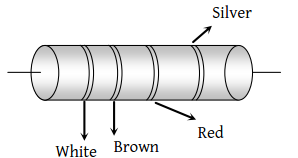The reciprocal of resistance is :

(1) Conductance

(2) Resistivity

(3) Voltage

(4) None of the above

Concept Questions :-

Derivation of Ohm's law
High Yielding Test Series + Question Bank - NEET 2020

Difficulty Level:

A solenoid is at the potential difference 60 V and current flows through it is 15 ampere, then the resistance of coil will be :

(1) 4 Ω

(2) 8 Ω

(3) 0.25 Ω

(4) 2 Ω

Concept Questions :-

Derivation of Ohm's law
High Yielding Test Series + Question Bank - NEET 2020

Difficulty Level:

All of the following statements are true except

(1) Conductance is the reciprocal of resistance and is measured in Siemens

(2) Ohm's law is not applicable at very low and very high temperatures

(3) Ohm's law is applicable to semiconductors

(4) Ohm's law is not applicable to electron tubes, discharge tubes and electrolytes

Concept Questions :-

Derivation of Ohm's law
High Yielding Test Series + Question Bank - NEET 2020

Difficulty Level:

The resistance of a discharge tube is :

(1) Ohmic

(2) Non-ohmic

(3) Both (1) and (2)

(4) Zero

Concept Questions :-

Derivation of Ohm's law
High Yielding Test Series + Question Bank - NEET 2020

Difficulty Level:

A steady current flows in a metallic conductor of non-uniform cross-section. The quantity/ quantities constant along the length of the conductor is/are :

(1) Current, electric field and drift speed

(2) Drift speed only

(3) Current and drift speed

(4) Current only

Concept Questions :-

Current and current density
High Yielding Test Series + Question Bank - NEET 2020

Difficulty Level:

σ1 and σ2 are the electrical conductivities of Ge and Na respectively. If these substances are heated, then

(1) Both σ1 and σ2 increase

(2) σ1 increases and σ2 decreases

(3) σ1 decreases and σ2 increases

(4) Both σ1 and σ2 decrease

Concept Questions :-

Derivation of Ohm's law
High Yielding Test Series + Question Bank - NEET 2020

Difficulty Level:

In the figure a carbon resistor has bands of different colours on its body as mentioned in the figure. The value of the resistance is(1) 2.2 kΩ

(2) 3.3 k kΩ

(3) 5.6 k kΩ

(4) 9.1 kΩ

Concept Questions :-

Colour coding of resistors
High Yielding Test Series + Question Bank - NEET 2020

Difficulty Level:

Masses of 3 wires of same metal are in the ratio 1 : 2 : 3 and their lengths are in the ratio 3 : 2 : 1. The electrical resistances are in ratio

(1) 1 : 4 : 9

(2) 9 : 4 : 1

(3) 1 : 2 : 3

(4) 27 : 6 : 1

Concept Questions :-

Derivation of Ohm's law
High Yielding Test Series + Question Bank - NEET 2020

Difficulty Level:

A wire of radius r has resistance R. If it is stretched to a radius of $\frac{3r}{4}$, its resistance becomes

(1) $\frac{9R}{16}$

(2) $\frac{16R}{9}$

(3) $\frac{81R}{256}$

(4) $\frac{256R}{81}$

Concept Questions :-

Derivation of Ohm's law
High Yielding Test Series + Question Bank - NEET 2020

Difficulty Level:

A copper wire has a square cross-section, 2.0 mm on a side. It carries a current of 8 A and the density of free electrons is 8 × 1028 m–3. The drift speed of electrons is equal to

(1) 0.156 × 10–3 m.s–1

(2) 0.156 × 10–2 m.s–1

(3) 3.12 × 10–3 m.s–1

(4) 3.12 × 10–2 m.s–1

Concept Questions :-

Current and current density